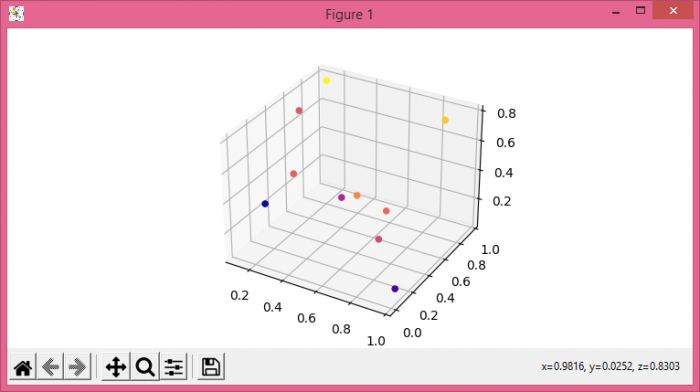# How to turn off transparency in Matplotlib's 3D Scatter plot?

To turn off transparency in Matplotlib's 3D scatter plot, we can use depthshade to shade the scatter markers to give the appearance of depth.

## Steps

• Set the figure size and adjust the padding between and around the subplots.
• Create a new figure or activate an existing figure.
• Add an ax to the figure as part of a subplot arrangement.
• Create random data points x, y and z using numpy.
• Use scatter method to plot x, y and z data points on 3D axes with depthshade=False.
• To display the figure, use show() methpod.

## Example

import numpy as np
from matplotlib import pyplot as plt

plt.rcParams["figure.figsize"] = [7.50, 3.50]
plt.rcParams["figure.autolayout"] = True

fig = plt.figure()

N = 10
x = np.random.rand(N)
y = np.random.rand(N)
z = np.random.rand(N)

ax.scatter(x, y, z, c=y, cmap='plasma', alpha=1, depthshade=False)

plt.show()

## Output